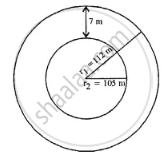# There is a Path of Uniform Width 7 M Round and Outside a Circular Garden of Diameter 210 M. Find the Area of the Path. - Mathematics

Sum

There is a path of uniform width 7 m round and outside a circular garden of diameter 210 m. Find the area of the path.

#### SolutionDiameter = 210 m

Radius of inner circle r= 105 m

Width = 7m

Radius of outer circle r1 = 105 + 7 = 112 m

∴ Area of path = pir_1^2 - pir_2^2

= pi[r_1^2 - r_2^2]

= 22/7(r_1 + r_2)(r_1 + r_2)

= 22/7 (112 + 105)(112 - 105)

= 22/7 xx 217 xx 7

= 4774 m2

Concept: Area of Circle
Is there an error in this question or solution?

#### APPEARS IN

Selina Concise Mathematics Class 8 ICSE
Chapter 20 Area of a Trapezium and a Polygon
Exercise 20 (D) | Q 9 | Page 234